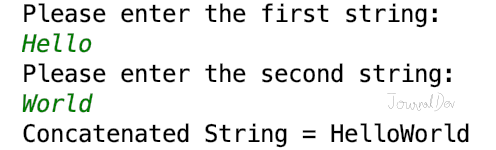# Python String Concatenation

Published on August 3, 2022By Pankaj
Developer and author at DigitalOcean.While we believe that this content benefits our community, we have not yet thoroughly reviewed it. If you have any suggestions for improvements, please let us know by clicking the “report an issue“ button at the bottom of the tutorial.

String Concatenation is a very common operation in programming. Python String Concatenation can be done using various ways. This tutorial is aimed to explore different ways to concatenate strings in a python program.

## Python String Concatenation

We can perform string concatenation using following ways:

• Using + operator
• Using join() method
• Using % operator
• Using format() function
• Using f-string (Literal String Interpolation)

### String Concatenation using + Operator

This is the most simple way of string concatenation. Let’s look at a simple example.

``````s1 = 'Apple'
s2 = 'Pie'
s3 = 'Sauce'

s4 = s1 + s2 + s3

print(s4)
``````

Output: `ApplePieSauce` Let’s look at another example where we will get two strings from user input and concatenate them.

``````s1 = input('Please enter the first string:\n')
s2 = input('Please enter the second string:\n')

print('Concatenated String =', s1 + s2)
``````

Output:

``````Please enter the first string:
Hello
World
Concatenated String = HelloWorld
``````It’s very easy to use + operator for string concatenation. However, the arguments must be a string.

``````>>>'Hello' + 4
Traceback (most recent call last):
File "<input>", line 1, in
TypeError: can only concatenate str (not "int") to str
``````

We can use str() function to get the string representation of an object. Let’s see how to concatenate a string to integer or another object.

``````print('Hello' + str(4))

class Data:
id = 0

def __init__(self, i):
self.id = i

def __str__(self):
return 'Data[' + str(self.id) + ']'

print('Hello ' + str(Data(10)))
``````

Output:

``````Hello4
Hello Data
``````

The biggest issue with + operator is that we can’t add any separator or delimiter between strings. For example, if we have to concatenate “Hello” and “World” with a whitespace separator, we will have to write it as `"Hello" + " " + "World"`.

### String concatenation using join() function

We can use join() function to concatenate string with a separator. It’s useful when we have a sequence of strings, for example list or tuple of strings. If you don’t want a separator, then use join() function with an empty string.

``````s1 = 'Hello'
s2 = 'World'

print('Concatenated String using join() =', "".join([s1, s2]))

print('Concatenated String using join() and whitespaces =', " ".join([s1, s2]))
``````

Output:

``````Concatenated String using join() = HelloWorld
Concatenated String using join() and spaces = Hello World
``````

### String Concatenation using % Operator

We can use % operator for string formatting, it can be used for string concatenation too. It’s useful when we want to concatenate strings and perform simple formatting.

``````s1 = 'Hello'
s2 = 'World'

s3 = "%s %s" % (s1, s2)
print('String Concatenation using % Operator =', s3)

s3 = "%s %s from JournalDev - %d" % (s1, s2, 2018)
print('String Concatenation using % Operator with Formatting =', s3)
``````

Output:

``````String Concatenation using % Operator = Hello World
String Concatenation using % Operator with Formatting = Hello World from JournalDev - 2018
``````

### String Concatenation using format() function

We can use string format() function for string concatenation and formatting too.

``````s1 = 'Hello'
s2 = 'World'

s3 = "{}-{}".format(s1, s2)
print('String Concatenation using format() =', s3)

s3 = "{in1} {in2}".format(in1=s1, in2=s2)
print('String Concatenation using format() =', s3)
``````

Output:

``````String Concatenation using format() = Hello-World
String Concatenation using format() = Hello World
``````

Python String format() function is very powerful, using it just for concatenation of strings is not its proper use.

### String Concatenation using f-string

If you are using Python 3.6+, you can use f-string for string concatenation too. It’s a new way to format strings and introduced in PEP 498 - Literal String Interpolation.

``````s1 = 'Hello'
s2 = 'World'

s3 = f'{s1} {s2}'
print('String Concatenation using f-string =', s3)

name = 'Pankaj'
age = 34
d = Data(10)

print(f'{name} age is {age} and d={d}')
``````

Output:

``````String Concatenation using f-string = Hello World
Pankaj age is 34 and d=Data
``````

Python f-string is cleaner and easier to write when compared to format() function. It also calls str() function when an object argument is used as field replacement.

## Conclusion

Python String formatting can be done in several ways. Use them based on your requirements. If you have to concatenate sequence of strings with a delimited, then use join() function. If some formatting is also required with concatenation, then use format() function or f-string. Note that f-string can be used with Python 3.6 or above versions.

You can checkout complete python script and more Python examples from our GitHub Repository.Pankaj

author

Developer and author at DigitalOcean.

#### Still looking for an answer?

Several of these examples of concatenation show no spaces between the two strings being concatenated (e.g s1 + s2, print(f’{name} age is {age} and d={d}'). However, in Python3, spaces are inserted between s1 and s2, and between the d= and the value of d).

- David

when i have to include age and name how can we concatenate using format()

- lavanya

hello, how to concatenate in the form: “Hello World” One line under the other

- bruno